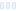# Thirst Equalizer

## Lemon Lime - EQ

Main info:

Thirst Equalizer
Lemon Lime - EQ
1 Serving
60 Calories
15 g
0 g
0 g

0 g
0 mg
0 g
15 mg
0 g
0 g

Percent calories from...
Nutrition Facts
For a Serving Size of (g)
How many calories are in Thirst Equalizer? Amount of calories in Thirst Equalizer: Calories Calories from Fat (%)
% Daily Value *
How much fat is in Thirst Equalizer? Amount of fat in Thirst Equalizer: Total Fat
How much sodium is in Thirst Equalizer? Amount of sodium in Thirst Equalizer: Sodium
How much potassium is in Thirst Equalizer? Amount of potassium in Thirst Equalizer: Potassium
How many carbs are in Thirst Equalizer? Amount of carbs in Thirst Equalizer: Carbohydrates
How many net carbs are in Thirst Equalizer? Amount of net carbs in Thirst Equalizer: Net carbs
How much fiber is in Thirst Equalizer? Amount of fiber in Thirst Equalizer: Fiber
How much glucose is in Thirst Equalizer? Amount of glucose in Thirst Equalizer: Glucose
How much protein is in Thirst Equalizer? Amount of protein in Thirst Equalizer: Protein
Vitamins and minerals
How much Vitamin A is in Thirst Equalizer? Amount of Vitamin A in Thirst Equalizer: Vitamin A
How much Vitamin C is in Thirst Equalizer? Amount of Vitamin C in Thirst Equalizer: Vitamin C
How much Calcium is in Thirst Equalizer? Amount of Calcium in Thirst Equalizer: Calcium
How much Iron is in Thirst Equalizer? Amount of Iron in Thirst Equalizer: Iron
Fatty acids
Amino acids
* The Percent Daily Values are based on a 2,000 calorie diet, so your values may change depending on your calorie needs.Loading similar foods...
Note: Any items purchased after clicking our Amazon buttons will give us a little referral bonus. If you do click them, thank you!Be cool

- Zen orangeI never skip arm day

- Buff broccoli